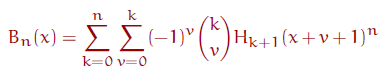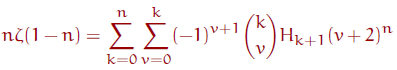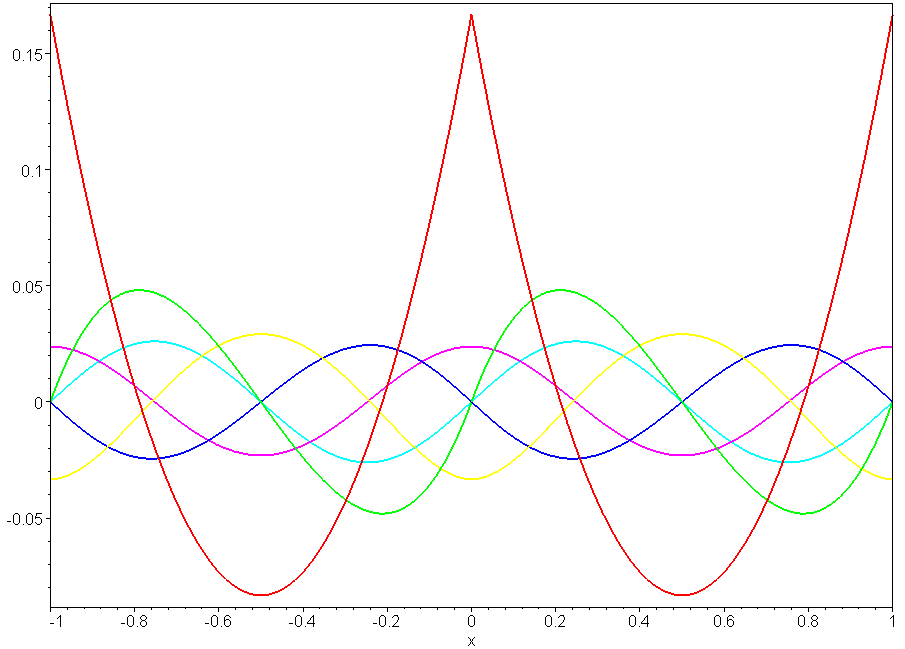﻿ A sequence transformation

## A sequence transformation and the Bernoulli numbers

#### Peter Luschny, April 2010

KEYWORDS: Bernoulli, Euler, Tangent, Harmonic, Binomial, Swiss-Knife, Worpitzky, Akiyama–Tanigawa.
Concerned with sequences: A027641, A027642, A131689, A019538, A028246, A141056, A027760, A176276, A176277.

### Contents

• 1 A sequence to sequence transformation
• 2 Bernoulli and Worpitzky numbers
• 3 Inverse polynomial harmony for Jakob
• 4 A primer on Worpitzky numbers
• 5 A challenger for the Akiyama–Tanigawa algorithm?
• 6 New sequences for the OEIS
• ### ::: A sequence to sequence transformation :::

Given an sequence f, an integer n ≥ 0 and a formal symbol x let me define a sequence of polynomials

T := proc(f,n,x) local k,v;
v=0..k), k=0..n) end:

 f1 xf1+f1−f2 x2f1+(2f1−2f2) x+2f3+f1−3f2 x3f1+(3f1−3f2)x2+(6f3+3f1 −9f2)x−6f4+12f3+f1−7f2 x4f1+(4f1−4f2)x3+(12f3 +6f1−18f2)x2 +(−24f4+48f3+4f1−28f2)x−60f4 +50f3+f1-15f2+24f5

The sequence of coefficients of these polynomials, sorted in descending order, is

 f1 f1 f1−f2 f1 2f1−2f2 f1−3f2+2f3 f1 3f1−3f2 3f1−9f2+6f3 f1−7f2+12f3−6f4 f1 4f1−4f2 6f1−18f2+12f3 4f1−28f2+48f3−24f4 f1−15f2+50f3−60f4+24f5

It is this sequence (the triangle read by rows) which I call the transform of f. f will usually be a sequence of integers or a sequence of rationals numbers. As a first check let us see  on which sequence the identity function f: x -> x is mapped.

11, -11, -2,  11, -3,  3,  -11, -4,  6,  -4,  11, -5, 10, -10,  5, -1

A signed Pascal triangle, no big surprise, but a good start. Now let us take some more interesting input sequences. What about f(n) the harmonic numbers?

H := proc(n) local i; add(1/i, i=1..n) end;
T(H, n, x) starts:
1
x - 1/2
x^2 - x + 1/6
x^3 - 3/2 x^2 + 1/2 x


We get the Bernoulli polynomials!

And feeding the transformation with twice the sum of the inverse powers of 2?

G := proc(n) local i; 2*add(1/2^i, i=1..n) end:
T(G, n, x) starts:
1
x - 1/2
x^2 - x
x^3 - 3/2 x^2 + 1/4


We get the Euler polynomials!

I give one more example, perhaps the most interesting one. Let

C := m -> if irem(m,4) = 0 then 0
else 1/((-1)^iquo(m,4)*2^iquo(m-1,2)) fi:

Interesting because the polynomials generated have integer coefficients:

               1
x
x^2  - 1
x^3  - 3 x
x^4  - 6 x^2  + 5
x^5  - 10 x^3  + 25 x
x^6  - 15 x^4  + 75 x^2  - 61

The Swiss knife polynomials!

If we evaluate these polynomials at 0 and 1 we get, respectively,

1, 0, -1,  0, 5, 0, -61, 0, 1385, 0, -50521, 0, 2702765, ...
1, 1, 0, -2, 0, 16, 0, -272, 0, 7936, 0, -353792, 0, ...


I am sure you know these sequences. For the purpose of reference I restyle the most important special case in math-parlance.

Let Bn(x) be the Bernoulli polynomials and Hn the harmonic numbers.I searched in the literature but could not find this formula. So I showed the formula in the newsgroup de.sci.mathematik; however, no one could provide a reference. If you know a reference for this formula, please let me know (the proof is easy once you see the formula on the blackboard...) .

I am amazed that this formula seems to be unknown. Much more exotic relations between the harmonic numbers and the Bernoulli numbers can be easily found (look for example at the section Assorted identities in the Wikipedia article). Indeed the formula looks like an exercise to the chapter Harmonic Summation in GKP's Concrete Mathematics (which is followed by the chapter Bernoulli numbers).

The case x = 1 can also be written with the Riemann zeta function asThis formula is valid for all n ≥ 0 provided for n = 0,1 the left hand side is understood as a limit value. By the way, this formula for the zeta values shows that the definition Bn = Bn(1) is to be preferred over the definition Bn = Bn(0) if you intend to go into the number theory business.On the other hand, some concrete mathematicians refrain from this definition being fearful to introduce thereby confusion to the world. Clearly this is a very strange argument since Jakob Bernoulli himself used B1 = 1/2 as you can see from the facsimile on Wikipedia, and Jakob was a very concrete mathematician.

### ::: Bernoulli and Worpitzky numbers :::

But this is not the end of the story. Looking at the table given above we can equate the constant coefficients of the polynomials with the Bernoulli numbers.

B_0 = 1*H(1);
B_1 = 1*H(1)-  1*H(2);                              [Table 1]
B_2 = 1*H(1)-  3*H(2)   +2*H(3);
B_3 = 1*H(1)-  7*H(2)  +12*H(3)-   6*H(4);
B_4 = 1*H(1)- 15*H(2)  +50*H(3)-  60*H(4)+  24*H(5);
B_5 = 1*H(1)- 31*H(2) +180*H(3)- 390*H(4)+ 360*H(5)- 120*H(6);


Now this is shocking: the coefficients on the right hand side of this table are the Worpitzky numbers; however, it is not Worpitzky's representation! Worpitzky gave the following representation with J(n) = 1/n.

B_0 = 1*J(1);
B_1 = 1*J(1)-  1*J(2);                               [Table 2]
B_2 = 1*J(1)-  3*J(2)   +2*J(3);
B_3 = 1*J(1)-  7*J(2)  +12*J(3)-   6*J(4);
B_4 = 1*J(1)- 15*J(2)  +50*J(3)-  60*J(4)+  24*J(5);
B_5 = 1*J(1)- 31*J(2) +180*J(3)- 390*J(4)+ 360*J(5)- 120*J(6);


So what happens if we expand table 1? We get yet another sum representation of the Bernoulli numbers, with even simpler coefficients!

B_0 = 1*J(1)
B_1 = 0*J(1)- 1*J(2)                                 [Table 3]
B_2 = 0*J(1)- 1*J(2)+  2*J(3)
B_3 = 0*J(1)- 1*J(2)+  6*J(3)-   6*J(4)
B_4 = 0*J(1)- 1*J(2)+ 14*J(3)-  36*J(4)+   24*J(5)
B_5 = 0*J(1)- 1*J(2)+ 30*J(3)- 150*J(4)+  240*J(5)-  120*J(6)


But wait a moment. Does table 1 and table 2 really amount to the same thing? Well, almost. In the first table B1 = −1/2 and in the second table B1 = 1/2. So the first case refers to a definition Bn = Bn(0) and the second case to a definition Bn = Bn(1). Apart from this the values in the tables are identical (as the two definitions differ only in this particular case).

Let us denote the coefficients in table 3 by V(n, k).

             V(n, k)
1
0, -1
0, -1, 2
0, -1, 6, -6
0, -1, 14, -36, 24
0, -1, 30, -150, 240, -120
0, -1, 62, -540, 1560, -1800, 720


What we have to find is a formula for the V(n, k). This is not difficult if we look at the relationship with the signed Worpitzky numbers W(n, k) = (-1)^k k! {n+1|k+1}. Here {n|k} denotes the Stirling set numbers (aka. Sn,k of the second kind). Our formal definition is V(n, k) = Sum(j=k..n) W(n,j). Now we can restate our findings:

 For n > 1 Bn = Sum(k=0..n) W(n, k) J(k+1)     = Sum(k=0..n) W(n, k) H(k+1)     = Sum(k=0..n) V(n, k)  J(k+1).

The first identity is Wropitzky's, which we take as granted. J(k+1) = H(k+1) − H(k).  So we can rewrite Wropitzky's sum as Sum(k=0..n) W(n, k)(H(k+1) − H(k)). Observe that Sum(k=0..n)  W(n, k) H(k) = 0,-1,0,0,0,0,0,.. (starting at n = 0). Thus if n <> 1 we can simplify to Sum(k=0..n) W(n, k) H(k+1), which is the second identity. The third identity follows from W(n, k) = V(n, k) − V(n, k+1).

For those who would like to check the formulas with Maple:

W := proc(n,k) (-1)^k*combinat[stirling2](n+1,k+1)*k! end:

seq(bernoulli(n, 1),n=0..23);

seq(bernoulli(n, 0),n=0..23);



### ::: Inverse polynomial harmony for Jakob :::

Putting the transformation into a plotting bag ...

MyPlot := proc(f,R) local T,i;
*f(k+1)*(x+v+1)^n,v=0..k),k=0..n) end:
plot([seq(T(f,i,x),i=2..7)],x=R,thickness=2,axes=boxed) end:


... we can now visualize the effect of the harmonic numbers versus the effect of the inverse numbers:

A := MyPlot(x->add(1/i, i=1..x),0..1):
B := MyPlot(x->1/x, -1..0):
plots[display]([A,B]);Be careful when interpreting this plot: these are not the Bernoulli polynomials. This are two plots in one. The left hand side (the interval [-1,0]) is generated by feeding J(n) into the transformation, the right hand side (the interval [0,1]) by feeding H(n) into the transformation. Only on [0,1] this coincides with the Bernoulli polynomials.

### ::: A primer on Worpitzky numbers :::

 Worpitzky (n ≥ 0 and k ≥ 0)  DefinitionW := proc(n, k) stirling2(n+1,k+1)*k! end: Recursion Wrec := proc(n, k) option remember; if k > n then 0 elif n = 0 then 1 else k*Wrec(n-1,k-1)+(k+1)*Wrec(n-1,k) fi end: Egfw := (x, y) -> exp(x)/(1+y*(1-exp(x))); V-Numbers (n ≥ 0 and k ≥ 0)   DefinitionV := proc(n, k) add(W(n, j), j=k..n) end: Recursion Vrec := proc(n, k) option remember; if k > n then 0 elif n = 0 then 1 else k*(Vrec(n-1, k-1) + Vrec(n-1, k)) fi end:  Egfv := (x, y) -> 1/(1+y*(1-exp(x)));

### ::: A challenger for the Akiyama–Tanigawa algorithm? :::

The Akiyama–Tanigawa algorithm is a cute way to compute the Bernoulli numbers.

AT := proc(n) local m, j, A;
for m from 0 by 1 to n do
A[m] := 1/(m + 1);
for j from m by -1 to 1 do
A[j - 1] := j * (A[j - 1] - A[j])
od od; A end:


The sum representation Bn = Sum(k=0..n) V(n, k)/(k+1) and the recursion for V(n,k) suggest a similar algorithm.

VB := proc(n) local m, j, A;
if n = 0 then 1 else A := 0; A := 1;
for m from 2 by 1 to n do A[m] := 0;
for j from m by -1 to 1 do
A[j] := j * (A[j - 1] + A[j])
od od;


An advantage of the VB algorithm over the AT algorithm is that it postpones rational arithmetic to the computation of the sum (the A[j]'s in AT are rational numbers, the A[j]'s in VB are integers).  Does this make a noticeable difference? Let us benchmark! I used the two procedures as given above; however, I replaced 'end:' by 'NULL; end:' to suppress the output. On my personal computer with Maple V Release 5 I got the following results (times in seconds):

 n AT VB STB 100 0.047 0.016 0.0 200 0.594 0.187 0.094 400 7.656 1.203 0.734 800 92.766 9.376 3.672

VB is up to ten times faster! This is fun. ;-) However, Maple's Bernoulli function is even faster. So I remembered the Knuth−Buckholz method, which is based on the computation of the tangent numbers (see CM, 6.5) and only needs a single division.

SeidelTangentBernoulli := proc(n) local i, j:
if n = 0 then 1 elif n = 1 then -1/2
elif n mod 2 = 1 then 0 else
i := 2^n; i := i*(i-1);
(-1)^iquo(n-1,2)*n*SeidelEulerTangent(n+1)/i fi end:


To compute the tangent numbers I used Seidel's boustrophedon transform (which is described on the Wikipedia page of the Bernoulli numbers, and of course in the paper by Millar, Sloane and Young, see example 3). The timings of the resulting algorithm are nearly the same as those of Maple's function (MV R5).

SeidelEulerTangent := proc(N) local A,Am,n,i,h,k,e,j;
n := 2*N - 5; if n < 0 then RETURN(1) fi;
h := iquo(n+1,4); A := array[-h..h];
A[-1] := 0; A := 1; k := 0; e := 1;
for i from 0 to iquo(n+1,2) do
Am := 0; A[k + e] := 0; e := -e;
for j from 0 to i do
Am := Am + A[k]; A[k] := Am; k := k + e;
od od; # print([seq(A[z], z=-h..h)]);
if N mod 2 = 0 then A[-h] else A[h] fi end:


### ::: New sequences for the OEIS :::

Consider the signless triangle Worpitzky(n, k)Harmonic(k) (which is also Abs(Stirling1(k+1,2)Stirling2(n+1,k+1))) for n ≥ 0 and k ≥ 0. The fact that the alternating sum of the rows is 0,1,0,0,0,... was a crucial step in some part of the proof above. This triangle should be supplemented by the sequence 0, 1, 3, 18, 125, 1020, 9667, 104790,.. which is the sum over the odd k's in a row. (Who comes up with a combinatorial interpretation?!) Note that the right hand side of the triangle is A000254 (unsigned Stirling numbers of first kind).

Based on the above considerations I submitted the following sequences to OEIS.

 A176276 Worpitzky(n, k)Harmonic(k), triangle read by rows.
 0, 0, 1, 0, 3, 3, 0, 7, 18, 11, 0, 15, 75, 110, 50, 0, 31, 270, 715, 750, 274, 0, 63, 903, 3850, 7000, 5754, 1764, 0, 127, 2898, 18711, 52500, 72884, 49392, 13068, 0, 255, 9075, 85470, 347550, 725004, 814968, 470448, 109584, 0, 511, 27990, 375155, 2126250, 6254598,
 OFFSET 0 REFERENCES LINKS Peter Luschny, A sequence transformation and the Bernoulli numbers . FORMULA T(n, k) = Abs(Stirling1(k+1, 2) Stirling2(n+1, k+1)). EXAMPLE 0, 0, 1, 0, 3, 3, 0, 7, 18, 11, 0, 15, 75, 110, 50, 0, 31, 270, 715, 750, 274, MAPLE T176276 := proc(n, k) local W, H; W := proc(n, k) stirling2(n+1, k+1)*k! end: H := proc(n) local i; add(1/i, i=1..n) end: # H(0) = 0 (empty sum convention) W(n, k)*H(k) end: CROSSREFS A176277, A028246. KEYWORD nonn, easy, tab

 A176277 Sum over the odd entries of the rows in the triangle Worpitzky(n, k)Harmonic(k) (A176276).
 0, 1, 3, 18, 125, 1020, 9667, 104790, 1281177, 17457840, 262493231, 4318429962, 77178551749, 1489209086820, 30859393432155, 683549418431934, 16118484827641841, 403156528379483160, 10661349675027656839, 297220064134533384306,
 OFFSET 0 COMMENT a(n) = sum_{k=0..n} (k mod 2) abs(Stirling1(k+1, 2) Stirling2(n+1, k+1)). REFERENCES LINKS Peter Luschny, A sequence transformation and the Bernoulli numbers . EXAMPLE Let W(n, k) the Worpitzky numbers and H(n) the harmonic numbers. a(3) = W(3,1)H(1)+W(3,3)H(3) =7*1+6*(11/6) = 18. MAPLE AA176277 := proc(n) local k; add((k mod 2)*T176276(n, k), k=0..n) end; CROSSREFS A176276, A028246. KEYWORD nonn, easy